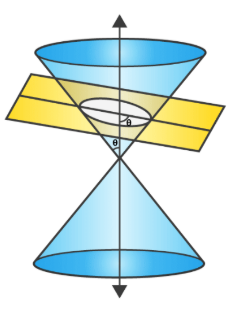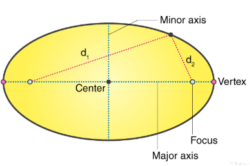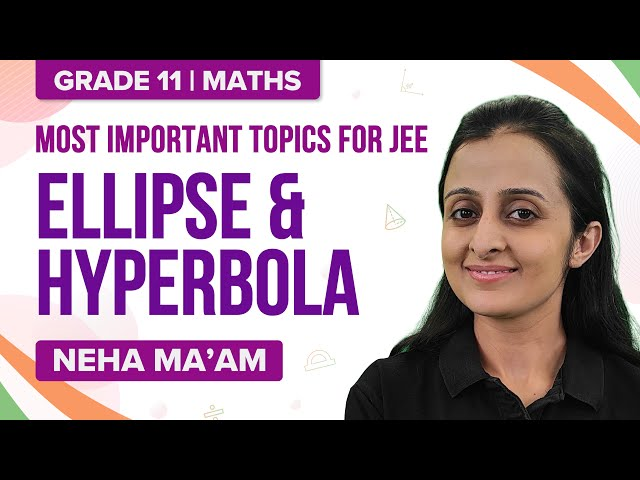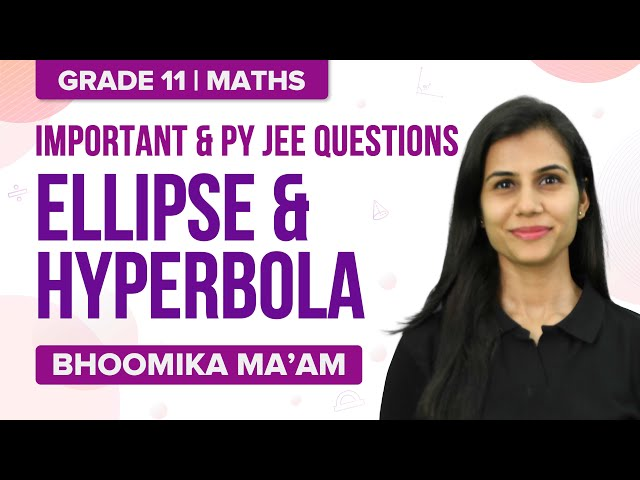# Basic Concepts of Ellipse

Ellipse is one of the conic sections which is obtained by the intersection of a right circular cone. An ellipse is the locus of a point traversing in a plane, such that the ratio of its distance from the fixed point and the line is a constant. It is always less than one. The eccentricity of an ellipse is e < 1. The general equation of a conic is ax2 + 2hxy + by2 + 2gx + 2fy + c = 0.

For example, If an egg is sliced in an oblique way, a curve can be seen by its edge.

The earth’s movement around the sun traces a similar but a bigger curve.

These curves can be termed as an ellipse.## General Definition of Ellipse

An ellipse is the locus of a point traversing in a plane such that the ratio of its distance from the fixed point and the line is a constant. It is always less than one. The eccentricity of an ellipse is e < 1. Read More

 The basic concepts of an ellipse involve: Focus: The fixed point on the ellipse. Directrix: The fixed straight line of the ellipse. Eccentricity: The constant ratio of the ellipse. Axis: The straight-line passing through the focus and perpendicular to the directrix. Vertex: The intersection points of the ellipse and the axis. Centre: It is the point which bisects every chord of the ellipse. Latus-Rectum: It is the chord passing through the focus and perpendicular to the axis. Major axis: The longest diameter is the major axis. The axis which passes through the centre and goes from one side of an ellipse to another, also the widest part of the ellipse. Minor axis: It is the shortest diameter that is present along the narrowest part of the ellipse. Focal chord: It is the chord passing through the focus of the ellipse. Focal distance: It is the distance from any point on the ellipse to the focus.## Notations and Standard Equation Of Ellipse

The representation of the different parts of the ellipse are as follows:

• The length of the major axis by ‘2a’.
• The length of the minor axis by ‘2b’.
• The distance between the foci by ‘2c’.
• The distance of focus from the centre is ‘c’.
• The relation between ‘a’, ‘b’, and ‘c’ is a2 = b2 + c2 or c2 = a2 – b2.

The standard equations of an ellipse is given by:

• $$\begin{array}{l}\frac{x^2}{a^2}+\frac{y^2}{b^2}=1\end{array}$$
• $$\begin{array}{l}\frac{x^2}{b^2}+\frac{y^2}{a^2}=1\end{array}$$

## Solved Ellipse Problems

Example 1: If the latus rectum of an ellipse is equal to half of its minor axis, then what is its eccentricity?

Solution:

2b2 / a = b

b / a = 1 / 2

b2 / a2 = 1 / 4

Hence e = √[1−b2 / a2] = √3 / 2

Example 2: Find the equation of the ellipse whose centre is at origin and which passes through the points (3, 1) and (2, 2).

Solution:

$$\begin{array}{l}\frac{x^2}{a^2}+\frac{y^2}{b^2}=1\end{array}$$
is the equation of the ellipse.

Since it passes through (3, 1) and (2, 2), so

$$\begin{array}{l}\frac{9}{a^2}+\frac{1}{b^2}=1\end{array}$$
and
$$\begin{array}{l}\frac{1}{a^2}+\frac{1}{b^2}=\frac{1}{4}\end{array}$$
$$\begin{array}{l}a^2=\frac{32}{3}\\b^2=\frac{32}{5}\end{array}$$

Hence required equation of ellipse is 3x2 + 5y2 = 32

Example 3: An ellipse is described by using an endless string which is passed over two pins. If the axes are 6 cm and 4 cm, then find the necessary length of the string and the distance between the pins in cm.

Solution:

Given 2a = 6, 2b = 4

i.e. , a = 3, b = 2

e2 = 1 − b2 / a2 = 5 / 9

e = √5 / 3

Distance between the pins = 2*a*e = 2√5 cm

Length of string = 2*a + 2*a*e = 6 + 2 √5 cm

Example 4: The locus of a variable point whose distance from (2, 0) is 2 / 3 times its distance from the line x = −[9 / 2] is an ellipse or a parabola. Check your answer.

Solution:

Let point P be (x1 , y1)

Consider √[(x1+ 2)2+ y12] = [2 / 3] (x1 + 9 / 2)

(x1 + 2)2 + y12 = [4 / 9] [(x1 + 9 / 2)2]

9 [x12 + y12 + 4x1 + 4] = 4 (x12 + 81 / 4 + 9x1 )

5x12 + 9y12 = 45

x12 / 9 + y12 / 5 = 1

Locus of (x1 ,y1) is x2 / 9 + y2 / 5 = 1, which is equation of an ellipse.

Example 5: What is the condition for the line lx + my− n=0 to be a tangent to the ellipse

$$\begin{array}{l}\frac{x^2}{a^2}+\frac{y^2}{b^2}=1\end{array}$$
?

Solution:

y = [−l / m]x + [n / m] is tangent to

$$\begin{array}{l}\frac{x^2}{a^2}+\frac{y^2}{b^2}=1\end{array}$$
, if

$$\begin{array}{l}\frac{n}{m}=\pm \sqrt{[b^2+a^2(l/m)^2]}\end{array}$$

Or

n2 = m2b2 + l2a2

### Ellipse and Hyperbola – Important Topics### Ellipse and Hyperbola – Important Questions## Frequently Asked Questions

### Give the standard equation of an ellipse.

The standard equation of an ellipse is given by (x2/a2) + (y2/b2) = 1.

### How do you denote the length of the major axis of an ellipse?

The length of the major axis of an ellipse is denoted by 2a.

### What do you mean by the focus of an ellipse?

Focus is the fixed point on the ellipse.

### How many latus rectum does an ellipse have?

An ellipse has two latus recta.

Test your Knowledge on concepts of ellipse